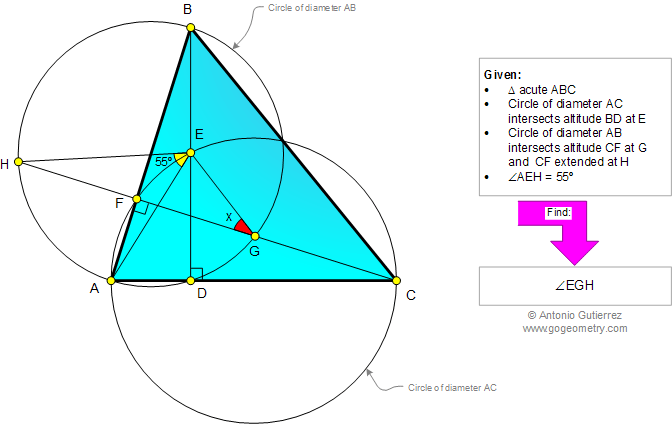# Online Geometry Problem 930: Acute Triangle, Altitudes, Circles, Diameter, Angle, Measure. Level: High School, SAT Prep, College, Mathematics Education

< PREVIOUS PROBLEM  |  NEXT PROBLEM >

 The figure below shows an acute triangle ABC. The circle of diameter AC intersects the altitude BD at E. The circle of diameter AB intersects the altitude CF at G and CF extended at H. If the measure of angle AEH is 55 degrees, find the measure of angle EGH.Home | Search | Geometry | Problems | All Problems | Open Problems | Visual Index | 10 Problems | 921-930 | Triangles | Circles | Angles | Altitude | Email | Solution / comment | By Antonio Gutierrez